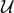Skip to main content

# Table 3 Table of notations

Symbol Meaning
b(l, m, u) The scheduling decision for the uth user in mth PRB in the lth subframe
b p(n) Total number of PRBs scheduled for device n over $$\mathcal {R}_{\mathrm {p}}$$
b np(n) Total number of PRBs scheduled for device n over $$\mathcal {R}_{\text {np}}$$
d(n) A function that measures channel quality difference for the nth device over the protected and non-protected PRBs
i v The number of active VUEs on the vth MN
j The index of an interferer
k The time index of received signal
l The index of a subframe
m The index of a PRB in a given subframe
n The index of an arbitrary device, i.e., either a UE or an MN, connecting to a macro cell
n (l, m) The device is selected to be scheduled at the mth PRB in the lth subframe in the macro cell
p The number of antennas equipped at the backhaul link of an MN
$$q_{n_{1}}$$ An arbitrary device served by macro BS sector 1
r(l, m, u) The throughput of the uth macro UE at the mth PRB in the lth subframe
r 0,i (v) The throughput of the access link of the vth MN when the ith subframe in the micro cells is configured as ABS
r 1,i (v) Throughput of the access link of the vth MN when the ith subframe in the micro cells is not configured as ABS
r p(n) The average rate of device n over $$\mathcal {R}_{\mathrm {p}}$$
r np(n) The average rate of device n over $$\mathcal {R}_{\text {np}}$$
s(n) The average throughput of device n, which can be either a macro UE or the backhaul link of an MN
s(u) The average throughput of the uth macro UE
s(v) The average throughput of the backhaul link of the vth MN
u The index of a macro UE
u (l, m) The macro UE that is selected to be scheduled at the mth PRB in the lth subframe
x k The desired signal transmitted at time k
x j, k The interfering signal from the jth interferer at time k
n 1 The index of a device served by macro BS sector 1
v The index of an MN
B p The size of $$\mathcal {R}_{\mathrm {p}}$$
B np The size of $$\mathcal {R}_{\text {np}}$$
G(ν) $$G(\nu)=N\,\frac {\nu }{\left (1+\nu \right)}$$ for ν≥0, a function defined to facilitate the solution of problem (7)
K A parameter used in the solution of (11), where K{0, 1, …, N−1} and G(d(K+1))≤KG(d(K))
L The number of total subframes in which the average throughput of a device in the macro cell is calculated
M The total number of PRBs in each subframe
N The total number of devices, i.e., N=N u+N m, connect to a macro cell
N 1 The number of devices served by macro BS sector 1
N c The number of subcarriers in an OFDMA system
N L The number of interferers experienced at the backhaul link of an MN
N m The number of MNs in a macro cell
N u The number of macro UEs in a macro cell
N sf The number of total subframes that average throughput target of the backhaul link of the MNs are calculated in the micro cells
$$\bar {R}(v)$$ The average throughput target of the backhaul link of the vth MN during N sf subframes
α A parameter used in the solution of (11), where α=λ pK
γ k The instantaneous output SINR at the MN backhaul receiver at time k
ε An averaging constant that is required in updating the average throughput of a given device
λ p A parameter used in the solution of (11), where λ p= max(G(d(K+1)), K)
λ np A parameter used in the solution of (11), where λ np=Nλ p
$$\sigma _{\mathrm {n}}^{2}$$ The noise power at each antenna
μ(l, u) The average throughput of user u
$$\mathcal {I}_{i}$$ $$\mathcal {I}_{i}\in \left \{ 0,\ 1\right \},\ i=1,\ 2,\ 3\ldots \,N_{\text {sf}}$$ indicate the status of the ABSs at the micro cellsThe sets denotes all the macro UEs in a cell
$$\mathcal {R}_{\mathrm {p}}$$ The set of protected PRBs in a macro cell
$$\mathcal {R}_{\text {np}}$$ The set of non-protected PRBs in a macro cell
$$\mathcal {S}_{1}$$ The set of users only scheduled in R p
$$\mathcal {S}_{2}$$ The set of users only scheduled in R np
$$\mathcal {S}_{3}$$ The set of users can be scheduled both in $$\mathcal {R}_{\mathrm {p}}$$and $$\mathcal {R}_{\text {np}}$$To denote uniform distributionThe sets denotes all the MNs in a cell
h k The channel vector of the desired signals at each reviver antenna of an MN at time k
h j, k The channel vector of the jth interfering signal observed at each reviver antenna of an MN at time k
n k The vector denotes the thermal noise at each receiver antenna of an MN at time k
w k The vector that contains the weight applied at each receiver antenna of an MN at time k
y k The vector that contains the received signal at each receiver antenna of an MN at time k
z k The combined signal at the output of an MN backhaul receiver at time k
R The noise covariance matrix of the reviver antennas of an MN
I p×p The identity matrix of size p
(·)H To denote the complex conjugate transpose of a matrix
EIGmax(·) To denote the dominant eigenvector of a matrix